How to Manually Work with Entity Numbers in Selections

June 4, 2014

In Part 1 of this blog series, I introduced how you can export a model M-file from COMSOL Multiphysics® simulation software to learn about the structure of the COMSOL Application Programming Interface (API). One important part of a model M-file is the selections that are made in order to set up properties for the domain, boundaries, etc. These selections are identified using numbers. Here, we explain how you can automate the handling of the entity numbers using LiveLink™ for MATLAB®.

Handling Selections When Changing the Geometry

When large changes are introduced to a model’s geometry, keeping track of the numbers that domains, boundaries, edges, or vertices are assigned is a challenge. These numbers are used to specify where certain settings should be applied: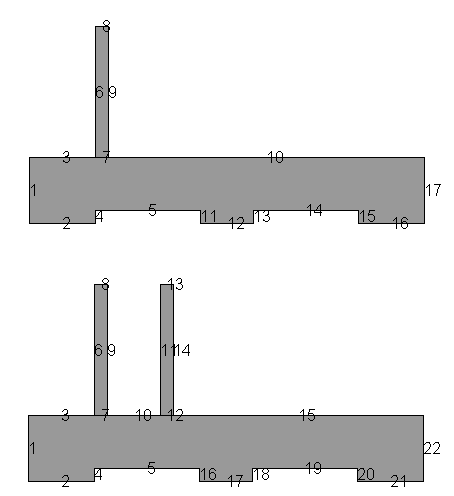The upper part of the above figure shows a heat sink with only one fin above the heat sink base. This is not a very efficient design, so I’ll add further fins in order to calculate the heat sink’s performance for different designs.

As you can see in the lower part of the figure, the numbering of the boundaries changes when a second fin is introduced on top of the heat sink base. Naturally, the boundaries that are a part of the newly introduced fin have to get numbers that are different from the previous design, since these boundaries have not been a part of the model so far. Introducing the new boundaries also means that some of the old numbers will change.

Note that the numbering is usually very difficult to predict, even for a simple 2D model like this. For a more complicated model in 2D and 3D, it becomes even more complicated. The examples and code used here could just as easily have been made with a 3D model, but I’ve chosen to go with a 2D model, as it becomes much easier to follow what’s going on.

When applying model settings to boundaries, the numbering becomes important. The figure below shows what the boundary condition for the cool side of the heat sink looks like: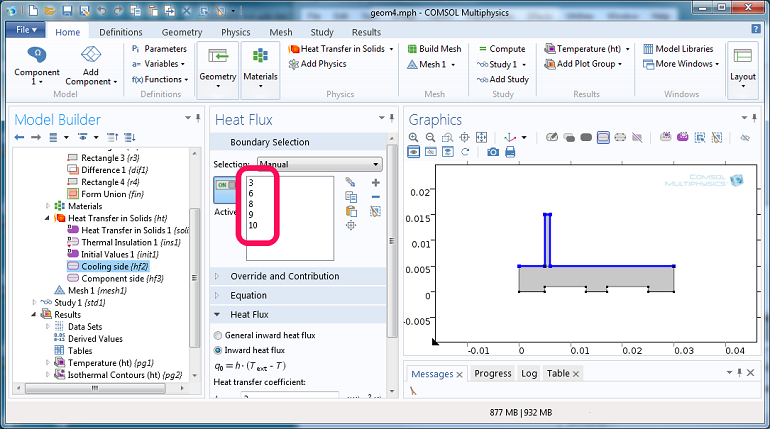The boundaries are easily identified in the Graphics window on the right. The corresponding numbers are seen in the Heat Flux settings window in the middle (circled in pink).

Selections in the Model M-file Code

Let’s have a look at what the corresponding selections look like in the model M-file code: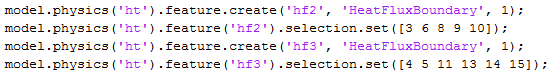The first set is for the upper part, where the cooling takes place, and the second set represents the hot part of the heat sink. When the second fin (or more, depending on your modeling scenario) is added to the model, we normally have little idea of which selection numbers to use.

There are two distinct ways of keeping track of the entity numbers:

1. Using LiveLink™ for MATLAB® functionality to track geometric entities based on their coordinates
2. Working with selections from geometric operations to track geometric entities

Method 1 is usually the easiest when you are introducing very large changes (such as new objects, for instance, like we will do here) into a geometry and the number of geometric entities change. This method makes it very easy to work with a model on the command line when changing the model bit by bit.

Method 2 usually requires more steps to set up, but has the added benefit that once the model has been set up, you can avoid the use of entity numbers altogether. The model can be used either via LiveLink™ for MATLAB® or directly in the COMSOL Multiphysics® user interface (UI), with ease.

Note that if you have a model where you don’t add or remove objects and you do not change its topology, then the numbering of the selections in the model will automatically be updated when you change model settings, and, for example, move objects from one location to another.

Coordinate Based Selections Using LiveLink™ for MATLAB® Functionality

The model is available as both an M-file and an MPH-file. Typically, the best solution is to use the MPH-file as a base for the changes to the model. Loading an MPH-file is usually faster than running the corresponding M-file. The MPH-file can, of course, contain meshes and solutions that can’t be saved as part of the M-file, which makes plotting model results a lot easier.

We load the model using this command: model = mphload('heat_param1')

A new fin is introduced into the model by these simple commands: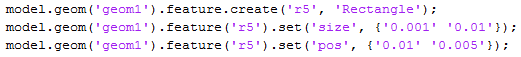The design is verified by using this command:

mphgeom(model,'geom1','edgelabels','on')

which plots the geometry in a MATLAB® figure.

This produces the lower part of the heat sink figure . In this figure, the numbers can be identified visually, but we would like to automate the process such that we can vary both the number of fins and their design. Getting the entity numbers of the boundaries that we need is easy if we use the mphselectbox command. This command selects entities using a box for which you have to specify the coordinates of two opposite corners. There is a corresponding function called mphselectcoords that selects entities inside a circle (or a sphere in 3D), but we will not use that function in this example.

Below, are the mphselectbox commands that we have to use for this model. For the cooling fins, we supply one set of coordinates that cover the entire upper part of the heat sink. This means that when I add more fins to the heat sink, these new fins will be covered by the rectangle and I can use the same code to get the selection numbers at that time. For the hot, lower part of the heat sink, I have to use two calls to mphselectbox and take the union of the selection numbers. “Union” is a standard MATLAB® function that takes the union of a set. It is also possible to use the MATLAB® functions, “setdiff” and “intersect”, in order to play around with the selection numbers to get the result you wish.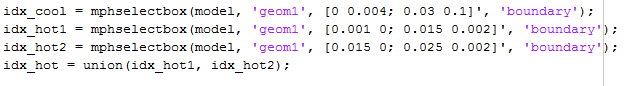The output looks like this: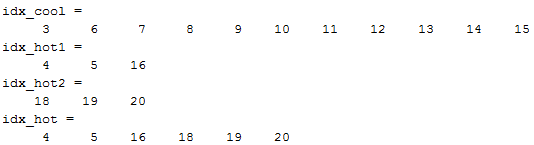Now it is easy to update the model settings with the correct numbers: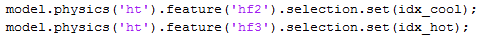Simulation

Once the selections have been set up, it is easy to adjust the number of fins. The following script shows how to set up a simple loop that will add four fins (one by one) to a model and solve. The goal is to find the effect of adding more fins to the heat sink.

The first line is used to load the model from an MPH-file. This way, we don’t have to run an M-file, which usually takes longer time than loading an MPH-file.

In the for loop, some more fins are added to the model with a proper size and location. The selection numbers are retrieved using mphselectbox and the model is solved. We generate a plot of each result to be studied when the analysis is completed.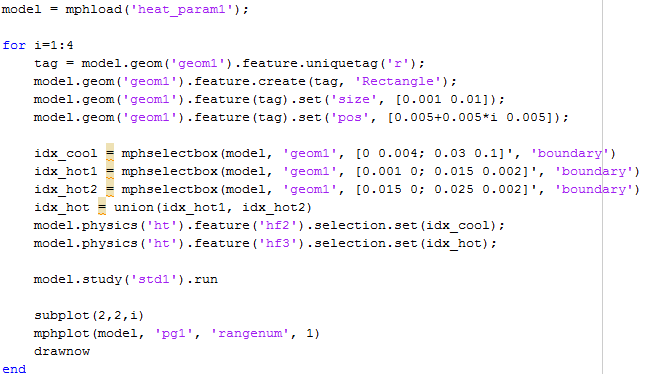The method “uniquetag” is used to get a tag for the new fin (Rectangle). When we use uniquetag, we don’t have to guess what tags are already taken and which might be available. This use of uniquetag with an ‘r’ as argument will return a tag that consists of ‘r’ and a number that is available.

What We Have Learned

When we change the geometry in a model (especially when we introduce new topology), it is important to keep track of the entity numbers for specifying model settings. An efficient way of doing this in a model M-file, or at the MATLAB® command line, is by using the wrapper functions, mphselectbox and mphselectcoords, that return entity numbers based on entity coordinates.

Next Up

The next blog post in this Working with M-files series will discuss how we can set up a model using selections from geometric operations. This leads to a model that is easy to handle both with LiveLink™ for MATLAB® and from within the COMSOL Multiphysics® user interface. Using this method means we are working directly with the selection objects and, in many cases, can avoid the use of entity numbers altogether.

Other Posts in This Series

MATLAB is a registered trademark of The MathWorks, Inc.

CategoriesAlain Glière
January 21, 2016

Hi Lars,

I would like to use the parameters of my geometry to create the select box. Instead of :
coordBox = [-300e-6 300e-6 ; -300e-6 300e-6 ; 0.49e-6 0.51e-6];
idx_ftri1 = mphselectbox(model, ‘geom1’, coordBox, ‘boundary’);

I would like to something like :
coordBox = [‘-wSi/2’ ‘wSi/2′;’-wSi/2′ ‘wSi/2’; ‘0.99*hGe’ ‘1.01*hGe’];

but it does not work. I also tried with cells without success.

Alain GlièreLars Gregersen
January 21, 2016

Hi Alain

Parameters defined in your Comsol model are not automatically known to Matlab.

If you wish to evaluate your expressions in Matlab you have to use the function mphevaluate that can calculate the value of your expressions (including unit if needed).Georgios Yiannakou
February 9, 2016

Hello, I have a geometry with 720 boundary sections, and I want to specify a different value of Magnetic Flux Density to each one ( I use mfnc physics interface). Is there any way of doing it, or is it possible to customise the name of the boundaries? Thanks.Ehsan Aalaei
May 14, 2019

HI Lars Gregersen

In my case, This method apparently is useless when two lines ( or the couple points of them) are in the same box. Do you have any suggestion for this problem?
one solution which comes into my mind was measuring the length of lines and get the desired line with specific length ( It’s possible using “if-condition” and measure function), but those two lines which are in the same box may be identical in length!Lars Gregersen
May 14, 2019

Hi Ehsan
I’m not really sure about what you have and what you seek. I interpret you post as: you have 2 vertices (in a box) and you wish to obtain the entity number of the edge between them. Perhaps you can use this example:
mphopen busbar
mphgeom(model,”,’vertexlabels’,’on’)
idx_edge=intersect(idx1,idx2)

If you don’t know the indices of your points, but instead know the coordinates then you should use mphselectcoords.

It should all be a matter of extracting the necessary indices and use Matlab’s set operations.Ehsan Aalaei
May 14, 2019

Thanks Lars for your consideration

In fact, I have the coordinates (and definitely their entity numbers) of two specific points (for instance the point 1 and 2) then, I’m gonna find the entity number of that edge which is made by those points (it might be a curve or even more complicated edge)

I don’t wanna use the “mphselectbox” or “mphselectcoords” because of this problem;

according to the schematic that I’ve created blew
Imagine in 2D, you have two lines and 3 points
(((( Line1 : between point1 and point2))) & (((Line2 : between point2 and point3)))
and you create a box using “mphselectbox” and the coordinates of the “point1” and “point2” ***to get the entity number of “line1″**. (actually,the entity number of “line1” is our goal)

as you see in this schematic, the result of “mphselectbox” function is the entity number of
“line1” and also “line2” !!!!!!!!!!!!
because “point3” is in this box (which is the smallest box contains “point1” and “point2”)

(point1) ———–(point3)
| \ |
| \ |
| \ | (Line2)
| (Line1) \ |
| \ |
| \ |
| \ |
|_ __ _ _ _ _ _ __(point2)

I have a complicated geometry with many points and edges in 3D and I’m gonna get the entity number of every edge using the start and end points of each edge.Ehsan Aalaei
May 14, 2019

(point1) ———–(point3)
|~~~~\~~~~~~|
|~~~~~\~~~~~|
|~~~~~~\~~~~| (line2)
|~~~(line1)\~~~|
|~~~~~~~~\~~|
|_ __ _ _ _ _ _ __(point2)Lars Gregersen
May 15, 2019

Thanks for you nice drawings 🙂
You could use mphgetadj as shown in the example above. If you have a lot of edges you wish to get the indices for, you may be better off using the functions getStartEnd and getAdj from the COMSOL API.Ehsan Aalaei
May 16, 2019

thank you, Lars
It works!!! 🙂
“getStartEnd” returns the start and end vertices (their IDs) of all edges in the first and second
row of the returned matrix. In addition, the number of columns indicate the entity number of related edge.

EXPLORE COMSOL BLOG